Next: AVO Analysis Before and Up: Results Previous: Results

Radon demultiple remains the default multiple suppression technique in many situations, particularly in 3-D, where acquisition sparsity may inhibit other techniques. On CMP gathers, primaries and multiples normally have different apparent velocities, and a Radon transform which sums across offset using various curvature parameters will focus the two types of events in different parts of the transform panel. The most natural curvature parameter for CMP data is the velocity of the hyperbola defined by the NMO equation () Foster and Mosher (1992). While the Hyperbolic Radon transform is a linear mapping, it is not time-invariant, and thus cannot be implemented efficiently as a Fourier domain operator. However, a multiple's residual moveout after NMO is approximately parabolic (quadratic) with offset, so a time-invariant Parabolic Radon transform is much faster, though not as accurate Hampson (1986); Kabir and Marfurt (1999).

To remove multiples, the primary energy in the transform panel is muted, and the inverse radon transform applied to produce an estimate of the multiples, which is subtracted from the data to produce a primary estimate. If we define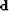as a raw CMP gather,as the linear mapping between radon transform space and data space,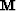as a mute operator that zeroes primary energy in radon transform space, and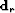as the estimated primaries, then we can express the radon demultiple process in equation form: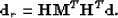(34)
Operatoris non-unitary (), so the amplitude of the estimated primaries will not match the recorded primaries. By casting Radon demultiple as a least-squares optimization problem, the Radon transform panel can be scaled such thatandare directly comparable. We first optimize a Radon transform panel,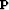, to minimize the data misfit: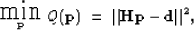(35)
and then apply the mute operator and adjoint ofto produce the estimated primaries:(36)
The finite frequency content of the data, limited extent of the array, and the intrinsic unresolvability of velocity information at zero offset all contribute to the non-uniqueness of the least-squares Radon demultiple problem. At far offsets, events with many zero-offset traveltimes and different velocities are fit equally well by a single curvature parameter. Low-frequency data makes moveout discrimination between multiples and primaries more difficult. At near offsets, all the events are fit equally well by all curvature parameters. All these pitfalls lead to reduced resolution of events in the Radon domain. So-called high resolution'' least-squares Radon transform implementations partially overcome these problems by imposing sparsity constraints in either the hyperbolic or parabolic Radon domain Sacchi and Ulrych (1995); Thorson and Claerbout (1985).

I implemented and tested least-squares Hyperbolic Radon demultiple (LSHRTD) on the CGG 3-D data subset. I performed 10 conjugate gradient iterations to produce an optimal, then applied a mute function which is zero for velocities greater than 90% known stacking velocity. The mute tapers linearly from 1.0 to 0.0 between 85% and 90% of the stacking velocity. The computational cost of LSHRTD is very similar to the cost of applying LSJIMP.

Figurecompares the results of applying LSJIMP and LSHRTD on a single CMP gather from the CGG 3-D data (CMPx=100, CMPy=4). The LSHRTD results are quite good, as we expect, given the high velocity gradient and relatively simple moveout seen in this region of the data. Spatial aliasing causes some artifacts on the LSHRTD primaries (Figure(b)), aroundseconds. The radon primaries seem to contain a bit more spatially uncorrelated noise than the LSJIMP primaries. LSJIMP is a more surgical'' separation technique, although the model regularization operators also exploit moveout differences to separate multiples and primaries.Figure 8
LSJIMP versus least-squares Hyperbolic Radon demultiple (LSHRTD) on one CMP gather of the CGG 3-D dataset. Panel (a): Raw data. Panel (b): LSHRTD estimated primaries. Panel (c): LSHRTD estimated multiples. Panel (d): LSJIMP estimated primaries. Panel (e): LSJIMP estimated multiples.

Figure, a stack of the LSHRTD estimated primaries, can be compared directly with the LSJIMP result, Figure. Like before, the multiples predominantly stack out, since the moveout separation is so significant. Still, a noticeable amount of multiple energy has been removed by LSHRTD, perhaps more than by LSJIMP. However, we immediately see some removed primary energy: for example, the strong primary near the bottom of the section.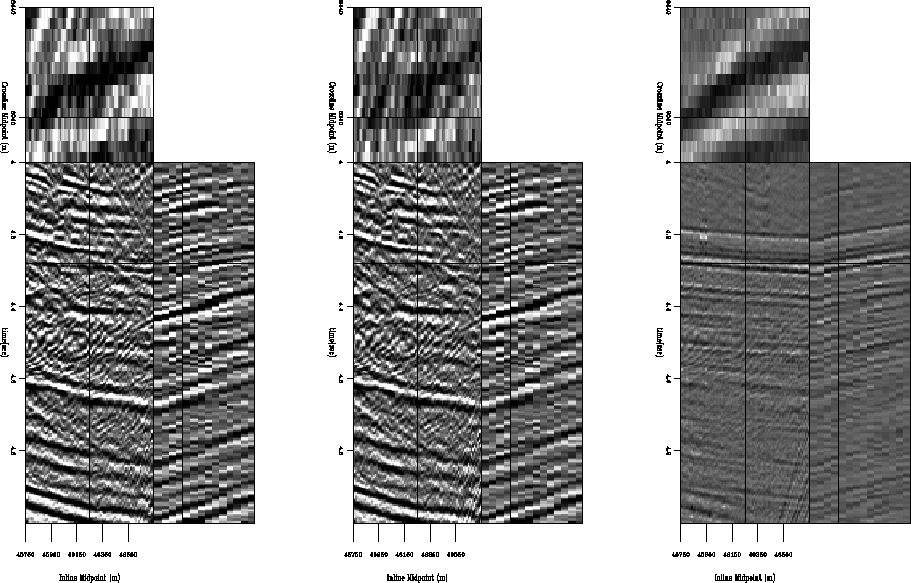NMO/Stack comparison before and after LSHRTD. Compare directly with Figure. Left: Raw data. Center: LSHRTD estimated primaries. Right: difference.